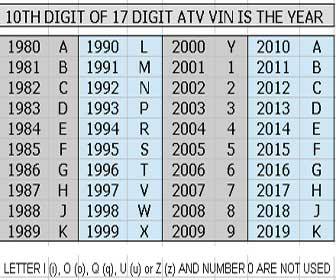# VIN Year Chart | Find and Decode Your Vehicle’s Year QuicklyLast updated:

## VIN Year Chart | Find and Decode Your Vehicle’s Year Quickly

Every vehicle produced since 1981 has a unique vehicle identification number, or VIN for short. A VIN contains data specific to the vehicle it is attached to including the year the vehicle was manufactured. The 10th digit of every VIN represents the year the vehicle was made. Here is a simple VIN year chart to help you decode the year your vehicle was manufactured in.The letters I (i), O (o), Q (q), U (u) and Z (z), as well as the number zero, are never used on a VIN because they can be confused with other digits. You may have noticed that some digits repeat, this is because the 10th digit of the VIN is re-used every 30 years.

## Complete 10th digit VIN year table

Match the 10th digit of your vehicle’s VIN to the VIN year table below to find out what year it was made. I hope you find this 10th digit VIN decoder chart useful. Learn more about your ATV VIN number.

Code Year Code Year Code Year Code Year Code Year
A = 1980 L = 1990 Y = 2000 A = 2010 L = 2020
B = 1981 M = 1991 1 = 2001 B = 2011 M = 2021
C = 1982 N = 1992 2 = 2002 C = 2012 N = 2022
D = 1983 P = 1993 3 = 2003 D = 2013 P = 2023
E = 1984 R = 1994 4 = 2004 E = 2014 R = 2024
F = 1985 S = 1995 5 = 2005 F = 2015 S = 2025
G = 1986 T = 1996 6 = 2006 G = 2016 T = 2026
H = 1987 V = 1997 7 = 2007 H = 2017 V = 2027
J = 1988 W = 1998 8 = 2008 J = 2018 W = 2028
K = 1989 X = 1999 9 = 2009 K = 2019 X = 2029